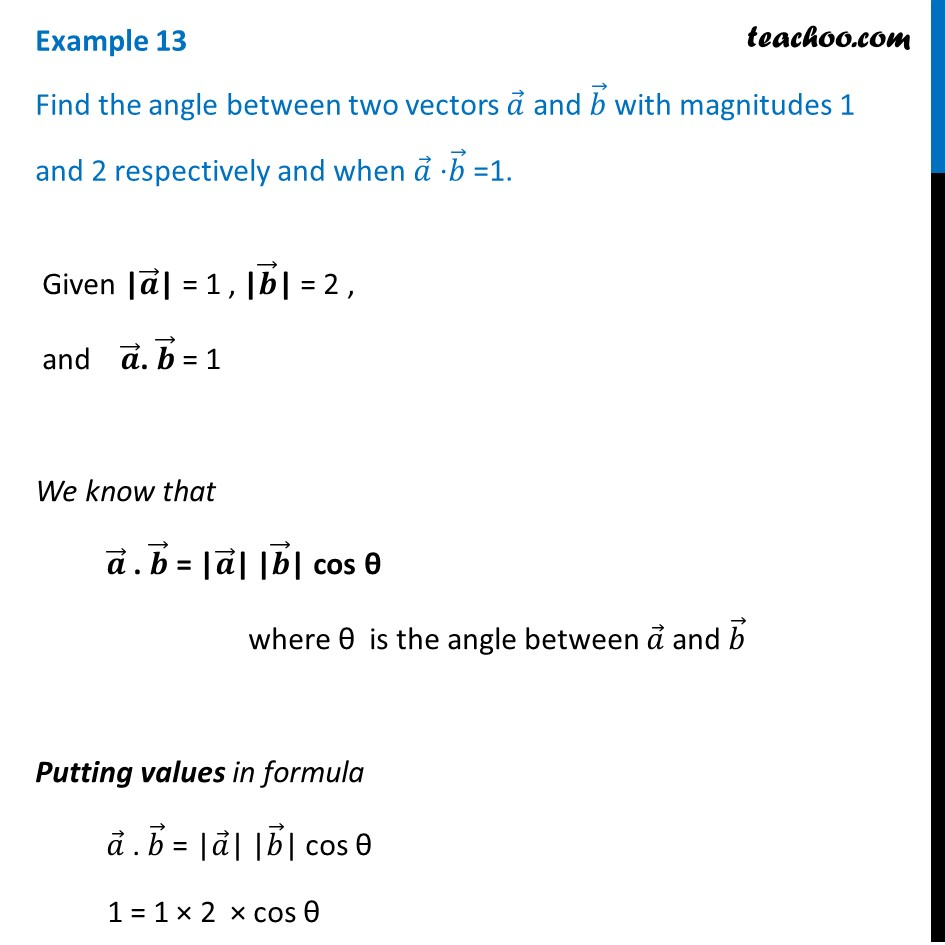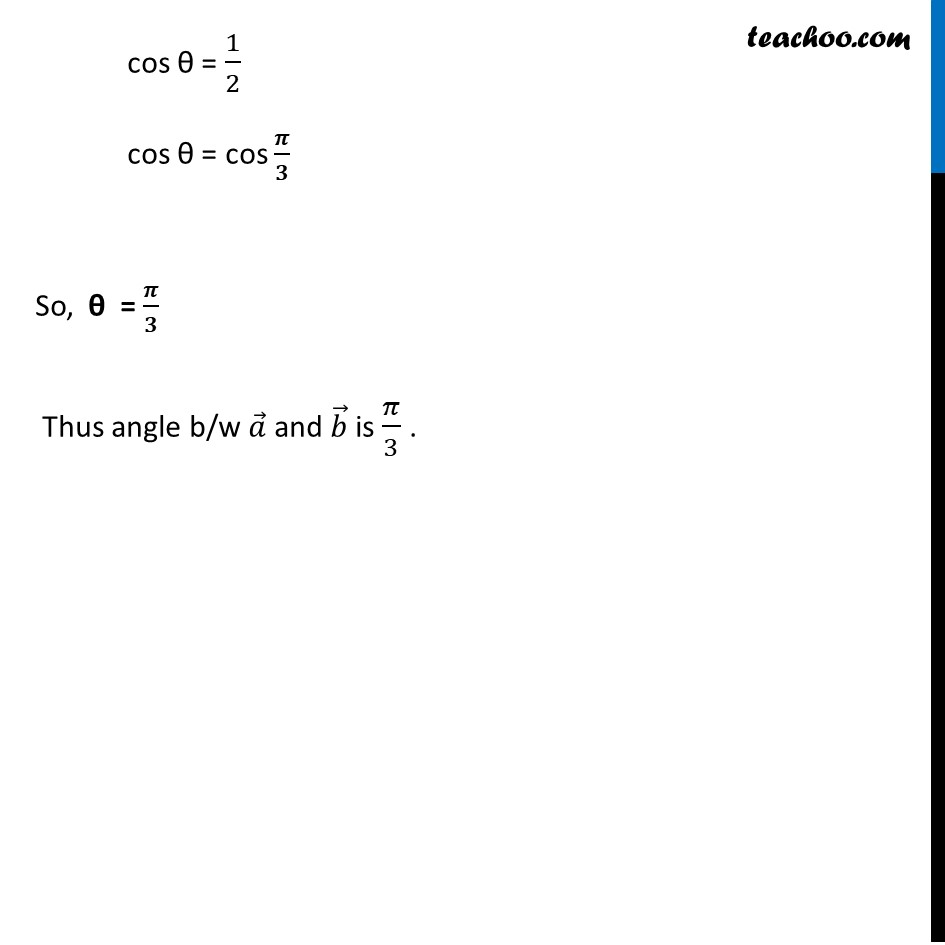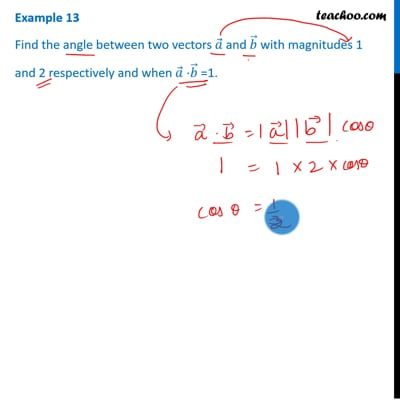Examples

Chapter 10 Class 12 Vector Algebra
Serial order wiseThis video is only available for Teachoo black users

Solve all your doubts with Teachoo Black (new monthly pack available now!)

### Transcript

Example 13 Find the angle between two vectors 𝑎 ⃗ and 𝑏 ⃗ with magnitudes 1 and 2 respectively and when 𝑎 ⃗ ⋅𝑏 ⃗ =1. "Given |" 𝒂 ⃗"| = 1 , |" 𝒃 ⃗"| = 2 , " "and " 𝒂 ⃗". " 𝒃 ⃗" = 1" We know that 𝒂 ⃗ . 𝒃 ⃗ = "|" 𝒂 ⃗"|" "|" 𝒃 ⃗"|" cos θ where θ is the angle between 𝑎 ⃗ and 𝑏 ⃗ Putting values in formula 𝑎 ⃗ . 𝑏 ⃗ = "|" 𝑎 ⃗"|" "|" 𝑏 ⃗"|" cos θ 1 = 1 × 2 × cos θ cos θ = 1/2 cos θ = cos 𝝅/𝟑 So, θ = 𝝅/𝟑 Thus angle b/w 𝑎 ⃗ and 𝑏 ⃗ is 𝜋/3 .